Publicité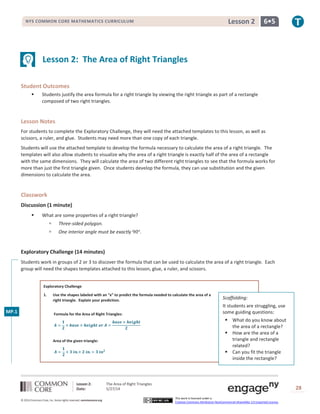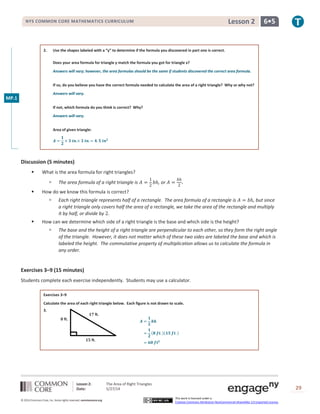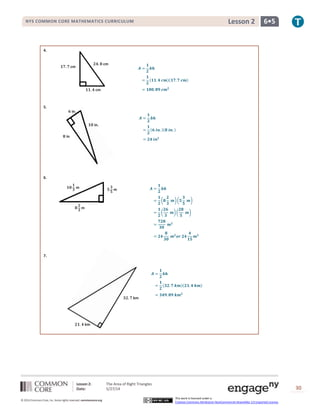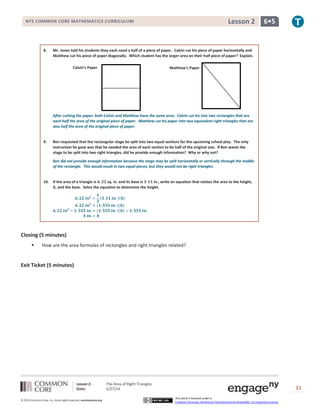Publicité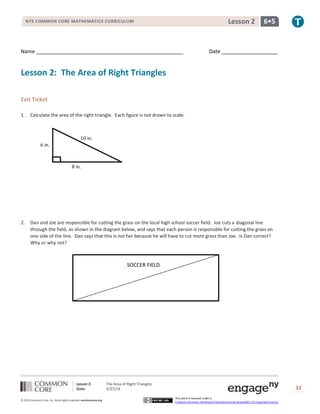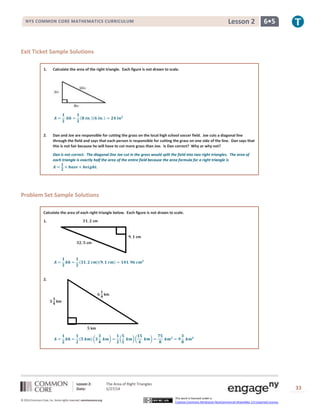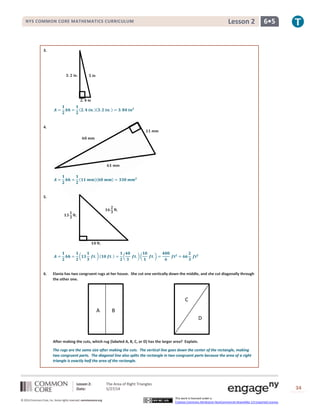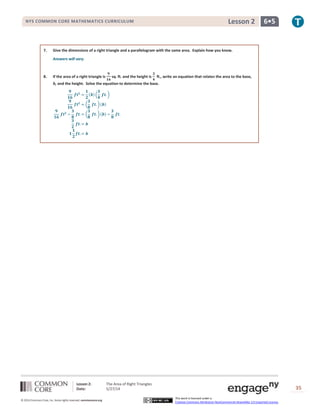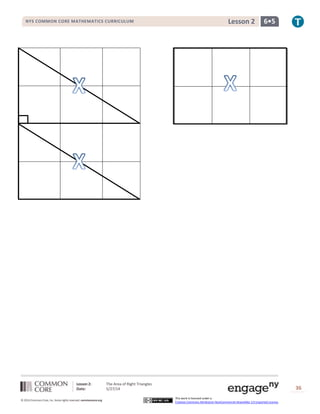Publicité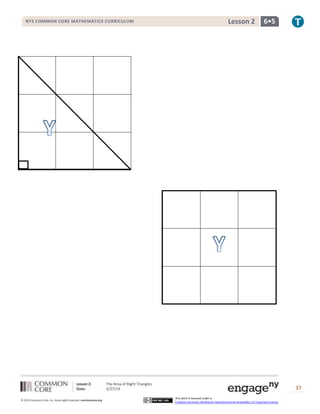Prochain SlideShare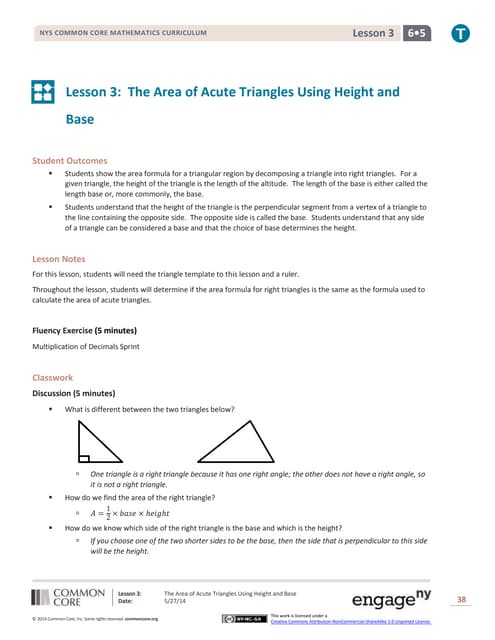G6 m5-a-lesson 3-t
Chargement dans ... 3
1 sur 10
Publicité

### G6 m5-a-lesson 2-t

1. Lesson 2: The Area of Right Triangles Date: 5/27/14 28 © 2014 Common Core, Inc. Some rights reserved. commoncore.org This work is licensed under a Creative Commons Attribution-NonCommercial-ShareAlike 3.0 Unported License. NYS COMMON CORE MATHEMATICS CURRICULUM 6•5Lesson 2 Lesson 2: The Area of Right Triangles Student Outcomes  Students justify the area formula for a right triangle by viewing the right triangle as part of a rectangle composed of two right triangles. Lesson Notes For students to complete the Exploratory Challenge, they will need the attached templates to this lesson, as well as scissors, a ruler, and glue. Students may need more than one copy of each triangle. Students will use the attached template to develop the formula necessary to calculate the area of a right triangle. The templates will also allow students to visualize why the area of a right triangle is exactly half of the area of a rectangle with the same dimensions. They will calculate the area of two different right triangles to see that the formula works for more than just the first triangle given. Once students develop the formula, they can use substitution and the given dimensions to calculate the area. Classwork Discussion (1 minute)  What are some properties of a right triangle?  Three-sided polygon.  One interior angle must be exactly 90°. Exploratory Challenge (14 minutes) Students work in groups of 2 or 3 to discover the formula that can be used to calculate the area of a right triangle. Each group will need the shapes templates attached to this lesson, glue, a ruler, and scissors. Exploratory Challenge 1. Use the shapes labeled with an “x” to predict the formula needed to calculate the area of a right triangle. Explain your prediction. Formula for the Area of Right Triangles: 𝑨 = 𝟏 𝟐 × 𝒃𝒂𝒔𝒆 × 𝒉𝒆𝒊𝒈𝒉𝒕 𝒐𝒓 𝑨 = 𝒃𝒂𝒔𝒆 × 𝒉𝒆𝒊𝒈𝒉𝒕 𝟐 Area of the given triangle: 𝑨 = 𝟏 𝟐 × 𝟑 𝒊𝒏.× 𝟐 𝒊𝒏. = 𝟑 𝒊𝒏 𝟐 MP.1 Scaffolding: It students are struggling, use some guiding questions:  What do you know about the area of a rectangle?  How are the area of a triangle and rectangle related?  Can you fit the triangle inside the rectangle?